## On a frictionless surface, a 32 kg student pushes a 43 kg student. If the 32 kg student slides back at 2.4 m/s, how fast will the 43 kg stud

Question

On a frictionless surface, a 32 kg student pushes a 43 kg student. If the 32 kg student slides back at 2.4 m/s, how fast will the 43 kg student be sliding and in what direction?

in progress 0
2 months 2021-07-31T19:17:34+00:00 1 Answers 2 views 0

The 43kg student will be sliding at 1.79m/s opposite the direction the 34kg student is going.

Explanation:

Conservation of linear momentum!

The law of conservation of momentum says that in an isolated system, the momentum before must equal the momentum after: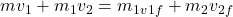.

For our two students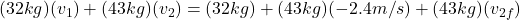(notice the – sign in -2.4m/s, this means going to the left)

since the students were not moving at first,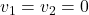, therefore we have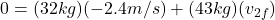solving for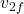gives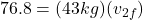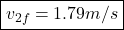Hence the 43kg student will be sliding at 1.79m/s to the right.